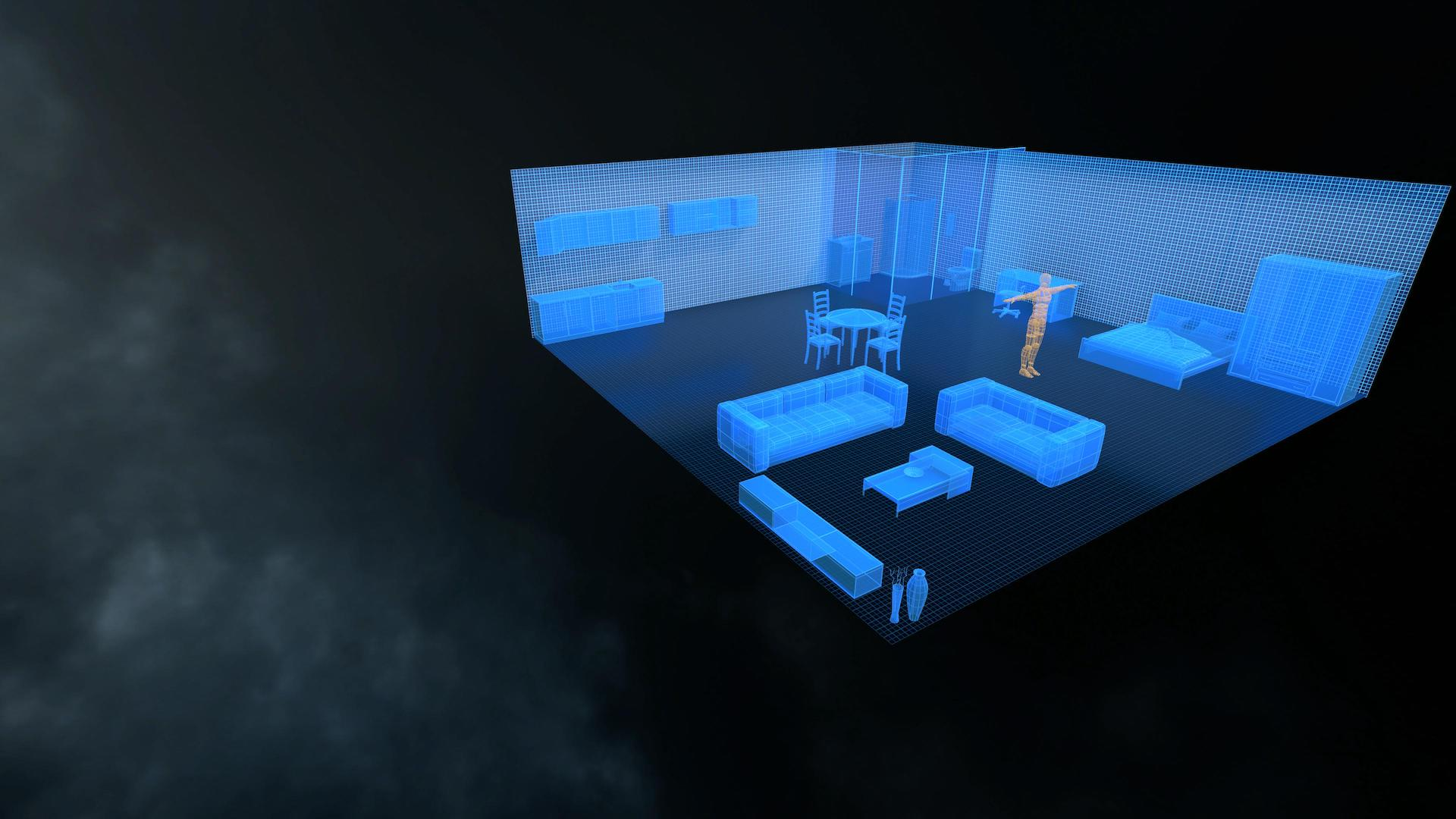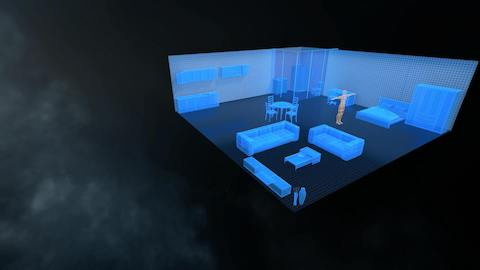Into the Matrix

Interactive and immersive lesson on the fundamentals of linear algebra.

### Sciences## Lesson Details

Enter the matrix to become immersed in vectors and vector operations. Interact with the mathematical concepts in an intuitive and engaging VR environment. Intrinsically abstract, the concepts of linear algebra have concrete interpretations in a physical 3Dspace.

Users can move around a virtual matrix using common linear algebra operations. Observe the spatial consequences of mathematical operations in solidifying understanding of linear algebra. Observe the relationship between maths and the objects in virtual space allowing interactive experimentation.

*In development - coming soon!

*Interested?

# Into the Matrix

Interactive and immersive lesson on the fundamentals of linear algebra.# Lesson Details:

Enter the matrix to become immersed in vectors and vector operations. Interact with the mathematical concepts in an intuitive and engaging VR environment. Intrinsically abstract, the concepts of linear algebra have concrete interpretations in a physical 3Dspace.

Users can move around a virtual matrix using common linear algebra operations. Observe the spatial consequences of mathematical operations in solidifying understanding of linear algebra. Observe the relationship between maths and the objects in virtual space allowing interactive experimentation.

*In development - coming soon!

*Interested?

Try It for Yourself!
We're happy to help with any questions you may have. Reach out to us!

## Into the Matrix